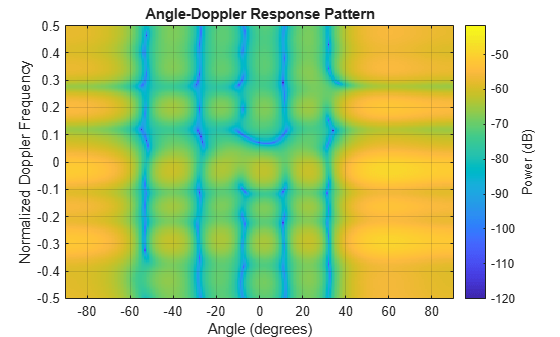# plotResponse

System object: phased.AngleDopplerResponse
Package: phased

Plot angle-Doppler response

## Syntax

```plotResponse(H,X) plotResponse(H,X,ELANG) plotResponse(H,X,PRF) plotResponse(___,Name,Value) hPlot = plotResponse(___) ```

## Description

`plotResponse(H,X)` plots the angle-Doppler response of the data in `X` in decibels. This syntax is available when the `ElevationAngleSource` property is `'Property'`.

The size of the first dimension of the input matrix can vary to simulate a changing signal length. A size change can occur, for example, in the case of a pulse waveform with variable pulse repetition frequency.

`plotResponse(H,X,ELANG)` plots the angle-Doppler response calculated using the specified elevation angle `ELANG`. This syntax is available when the `ElevationAngleSource` property is `'Input port'`.

`plotResponse(H,X,PRF)` plots the angle-Doppler response calculated using the specified pulse repetition frequency `PRF`. This syntax is available when the `PRFSource` property is ```'Input port'```.

`plotResponse(___,Name,Value)` plots the angle-Doppler response with additional options specified by one or more `Name,Value` pair arguments.

`hPlot = plotResponse(___)` returns the handle of the image in the figure window, using any of the input arguments in the previous syntaxes.

## Input Arguments

 `H` Angle-Doppler response object. `X` Input data. `ELANG` Elevation angle in degrees. Default: Value of `Elevation` property of `H` `PRF` Pulse repetition frequency specified as a positive scalar. To enable this argument, set the `PRFSource` property to ```'Input port'```. Units are in Hertz.

### Name-Value Pair Arguments

Specify optional comma-separated pairs of `Name,Value` arguments. `Name` is the argument name and `Value` is the corresponding value. `Name` must appear inside quotes. You can specify several name and value pair arguments in any order as `Name1,Value1,...,NameN,ValueN`.

 `'NormalizeDoppler'` Set this value to `true` to normalize the Doppler frequency. Set this value to `false` to plot the angle-Doppler response without normalizing the Doppler frequency. Default: `false` `'Unit'` The unit of the plot. Valid values are `'db'`, `'mag'`, and `'pow'`. Default: `'db'`

## Examples

expand all

Plot the angle-Doppler response of the 190th cell of a collected data cube.

```load STAPExampleData; x = shiftdim(STAPEx_ReceivePulse(190,:,:)); hadresp = phased.AngleDopplerResponse(... 'SensorArray',STAPEx_HArray,... 'OperatingFrequency',STAPEx_OperatingFrequency,... 'PropagationSpeed',STAPEx_PropagationSpeed,... 'PRF',STAPEx_PRF); plotResponse(hadresp,x,'NormalizeDoppler',true);```